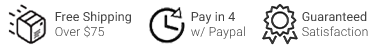Slideshow Items

Buchla & Tiptop Audio

# Model 257t

\$165.00
Available Immediately
Product number: CV4260Dual Voltage Processor

• +12V mA mA mA HP mm

## Dual Control Voltage Processor

Model 257 Dual Control Voltage Processor consists of two identical sections, each of which permits several applied control voltages to define a single output voltage according to the equation:

(Va * K) + ((Vb * (1-M)) + (Vc * M)) + Voffset = V_out

The algebraic manipulations possible with this module include addition, subtraction, scaling, inversion, and multiplication. Also incorporated is the capability of using one control voltage (M) to transfer control from one applied voltage (V_b) to another (V_c).

Functions

Attenuverter
Va is the voltage input a for attenuverter (left jack on the panel)
K knob can scale the incoming Va voltage either positively or negatively

Vb is the voltage input b for the left side of the crossfader (second jack from the left) +.5 switch adds 5 volts to the input of Vb
Vc is the voltage input c for the right side of the crossfader (fourth jack from the left)
M scales Vb from 1 to 0 based on the position of M knob
M scales Vc from 0 to 1 based on the position of M knob
The middle CV input at M/M can crossfade between Vb and Vc (third jack from the left) The M knob and M CV added together to control crossfade position

Offset
Voffset Adds 0 - 10 volts to any combination of Va + Vb + Vc

Output
Vout is the combined voltage of the attenuverter, crossfader and offset (right jack on the panel)